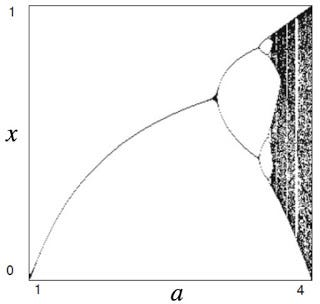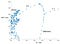# A Bifurcation Diagram for the Heart

In Figure 10.26 of Intermediate Physics for Medicine and Biology, Russ Hobbie and I plot a bifurcation diagram for the logistic map: xj+1 = a xj (1 − xj).A bifurcation diagram for the logistic map, showing 300 values of xj for values of a between 1 and 4. Figure 10.26 in Intermediate Physics for Medicine and Biology.

The bifurcation diagram summarizes the behavior of the map as a function of the parameter . Some values of a correspond to a steady state, others represent period doubling, and still others lead to chaos.

When I teach Biological Physics, I don’t introduce chaos using the logistic map. Instead, I solve IPMB’s Homework Problem 41, about cardiac restitution and the onset of fibrillation.

While Problem 41 provides insight into chaos and its relation to cardiac arrhythmias, Russ and I don’t draw a bifurcation diagram that summarizes how the action potential duration, APD, depends on the cycle length, CL (the time between stimuli, it’s the parameter analogous to a in the logistic map). In this post I present such a diagram.A bifurcation diagram associated with Homework Problem 41 in Intermediate Physics for Medicine and Biology. The plot shows 20 values of APDj for values of CL between 100 and 400 ms.

I don’t have the software to create a beautiful diagram like in Fig. 10.26, so I made one using MATLAB. It doesn’t have as much detail as does the diagram for the logistic map, but it’s still helpful.

The region marked 1:1 (for CL = 310 to 400 ms) implies steady-state behavior: Each stimulus excites an action potential with a fixed duration. Transients existed before the system settled down to a steady state, so I discarded the first 10,000 iterations before I plotted 20 values of ( j = 10,001 to 10,020).

Between CL = 283 and 309 ms the system predicts alternans: the response to the stimulus alternates between two APDs (long, short, long, short, etc.). Sometimes this is called a 2:2 response. Alternans are occasionally seen in the heart, and are usually a sign of trouble.

From CL = 154 to 282 ms the response is 2:1, meaning that after a first stimulus excites an action potential the second stimulus occurs during the refractory period and therefore has no effect. The third stimulus excites another action potential with the same duration as the first (once the transients die away). This is a type of period doubling; the stimulus has period CL but the response has period 2 CL. In cardiac electrophysiology, this behavior resembles second-degree heart block.

For a CL of 153 ms or shorter, the system is chaotic. I didn’t explore the diagram in enough detail to tell if self-similar regions of steady-state behavior exist within the chaotic region, as occurs for the logistic map (see Fig. 10.27 in IPMB).

A bifurcation diagram is a useful way to summarize the behavior of a nonlinear system, and provides insight into deadly heart arrhythmias such as ventricular fibrillation.

Originally published at http://hobbieroth.blogspot.com.

Professor of Physics at Oakland University and coauthor of the textbook Intermediate Physics for Medicine and Biology.

## More from Brad Roth

Professor of Physics at Oakland University and coauthor of the textbook Intermediate Physics for Medicine and Biology.